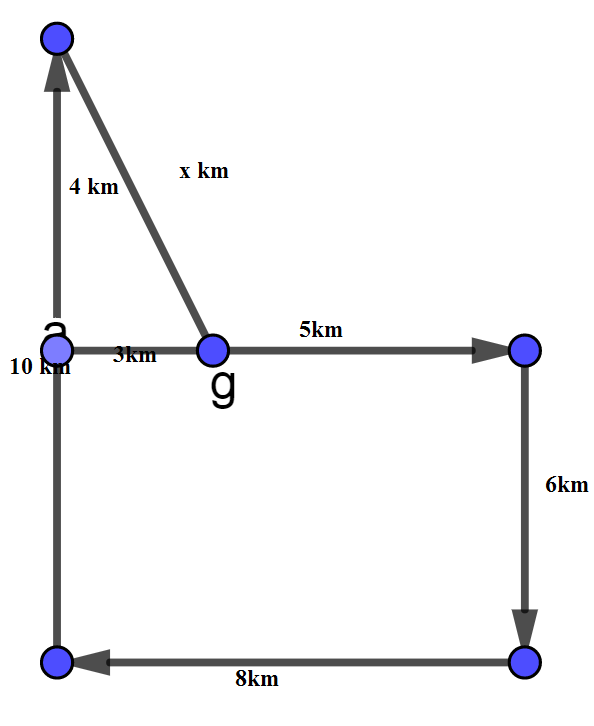Question 4

# A farmer is preparing his field. He starts his tractor from garage and drives 5 km towards East. From there, he turns right and drives 6 km. Then, he turns right and drives 8 km. Again, he turns right and drives 10 km when his tractor breaks down. What is the shortest distance between his current location and garage?

Solution

From the given question we draw the  draw the diagram is given belowFrom the above diagram

$$x^2 = 4^2 + 3^2$$

$$\Rightarrow x^2 = 16+9$$

$$\Rightarrow x = \sqrt{25}$$

$$\Rightarrow x = 5 km$$ Ans